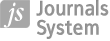ORIGINAL PAPER
Effect of Capillarity on Fourth Order Nonlinear Evolution Equation for Two Stokes Wave Trains in Deep Water in the Presence of Air Flowing Over Water
,

More details
Hide details
1
Department of Mathematics Indian Institute of Engineering Science and Technology, Shibpur P.O- B. Garden, Shibpur, Dist.- Howrah- 711103 West Bengal, INDIA

2
Department of Mathematics, Kandi Raj High School P.O.- Kandi, Dist.- Murshidabad West Bengal, INDIA

Online publication date: 2015-05-23

Publication date: 2015-05-01

International Journal of Applied Mechanics and Engineering 2015;20(2):267–282

KEYWORDS
ABSTRACT
Fourth order nonlinear evolution equations, which are a good starting point for the study of nonlinear water waves, are derived for deep water surface capillary gravity waves in the presence of second waves in which air is blowing over water. Here it is assumed that the space variation of the amplitude takes place only in a direction along which the group velocity projection of the two waves overlap. A stability analysis is made for a uniform wave train in the presence of a second wave train. Graphs are plotted for the maximum growth rate of instability wave number at marginal stability and wave number separation of fastest growing sideband component against wave steepness. Significant improvements are noticed from the results obtained from the two coupled third order nonlinear Schrödinger equations.

REFERENCES (15)
1.
Benjamin T.B. and Feir J.E (1967): The disintegration of wave trains on deep water. Part I Theory. - J. Fluid Mech., vol.27, pp.417-430.

2.
Debsharma S. and Das K.P. (2002): Fourth order non linear evolution equation for counter propagating capillary gravity wave packets on the surface of water of infinite depth. - Physics of Fluids, vol.14, pp.2225-2234.

3.
Debsharma S and Das K.P. (2005): A higher order non linear evolution equation for broader bandwidth gravity waves in deep water. - Physics of Fluids, vol.17, pp.104101-104108.

4.
Debsharma S and Das K.P. (2007): Fourth order non linear evolution equation for capillary gravity wave packets in the presence of another wave packet in deep water. - Physics of Fluids, vol.19, pp.1-16.

5.
Dhar A.K and Das K.P. (1990): Fourth order nonlinear evolution equation for deep water surface gravity waves in the presence of wind blowing over water. - Phys. Fluids A2(5), pp.778-783.

6.
Dhar A.K. and Das K.P. (1991): Fourth order nonlinear evolution equation for two Stokes wave trains in deep water. - Phys. Fluids A3(12), pp.3021-3026.

7.
Dhar A.K. and Das K.P. (1994): Stability analysis from fourth order evolution equation for small but finite amplitude interfacial waves in the presence of a basic current share. - J. Austral. Math. Soc.B35, pp.348-365.

8.
Dysthe K.B. (1979): Note on a modification to the nonlinear Schrödinger equation for application to deep water waves. - Proc. R. Soc. Lond.A369, pp.105-114.

9.
Hogan S.J. (1985): The fourth order evolution equation for deep water gravity capillary waves. - Proc. R. Soc. Lond. A402, pp.359-372.

10.
Janssen P.A.E.M. (1983): On fourth order evolution equation for deep water waves. - J. Fluid Mech., vol.126, pp.1-11.

11.
Longuet-Higgins M.S. (1987): The instabilities of gravity waves of finite amplitude in deep water. - I. Super Harmonics. Proc. R. Soc. Lond. A360, pp.417-488.

12.
Longuet-Higgins M.S. (1978): The instabilities of gravity waves of finite amplitude in deep water. - II. Sub Harmonics. Proc. R. Soc. Lond. A360, pp.489-506.

13.
Majumder D.P. and Dhar A.K. (2009): Stability analysis from fourth order evolution equation for two stokes wave trains in deep water in the presence of air flowing over water. - International Journal of Applied Mechanics and Engineering, vol.14, pp.989-1008.

14.
Majumder D.P. and Dhar A.K. (2011): Stability of small but finite amplitude interfacial capillary gravity waves for perturbations in two horizontal directions. - International Journal of Applied Mechanics and Engineering, vol.16, pp.425-434.

15.
Roskes G.J. (1976): Nonlinear multiphase deep-water wave trains. - Phys. Fluids, vol.19, pp.1253-1254.

 eISSN: 2353-9003 ISSN: 1734-4492